Elite indicators - metatrader 5 version :) - page 7373

Usdx

airquest

Thanks for the "trick"The only problem is that we have to do it manually (and I am a lazy man)

Will see what can be done regarding USD index to make a reasonably useful indicator : they removed almost every function that allowed us simple synchronization of 2 different symbols in metatrader 5 so it seems that all has to be done from the scratch

No need to worry about my request anymore, Mladen. I found a broker that has MT5 with USD Index (Liteforex). Should have thought about that before asking. Thanks.139679

This is a rsi adaptive T3 indicator for metatrader 5. It uses the T3 ability to produce smooth result and to still be adaptive to the market conditions changes (so to "speed up" or to "slow down" when necessary depending on price changes)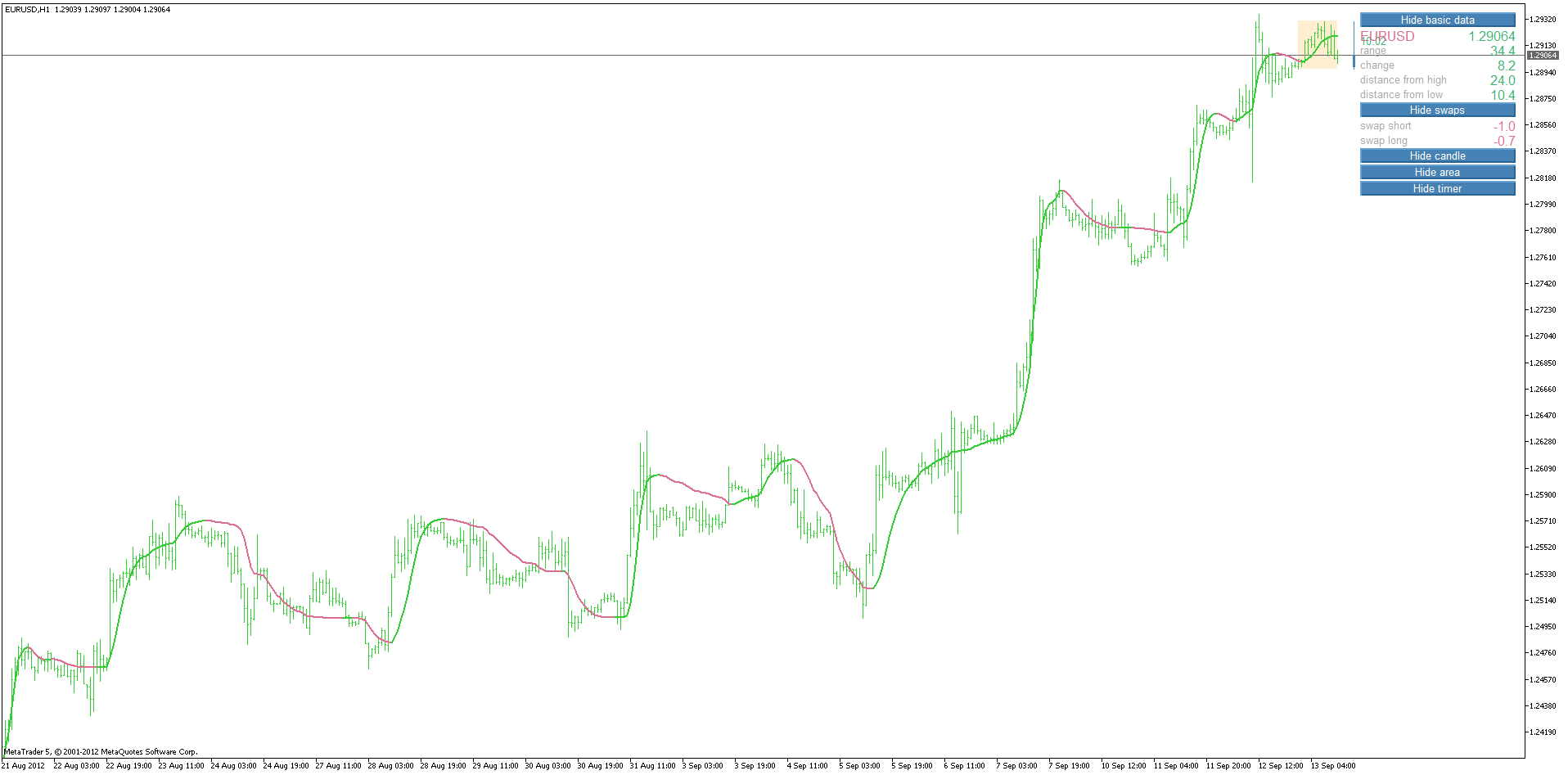Files:139679

High low activator ...

A variation of the Gann High Low activator which filters out a lot of false signals compared to the original calculation (it turns out to be quite good in strong trends and is best to use on higher time frames )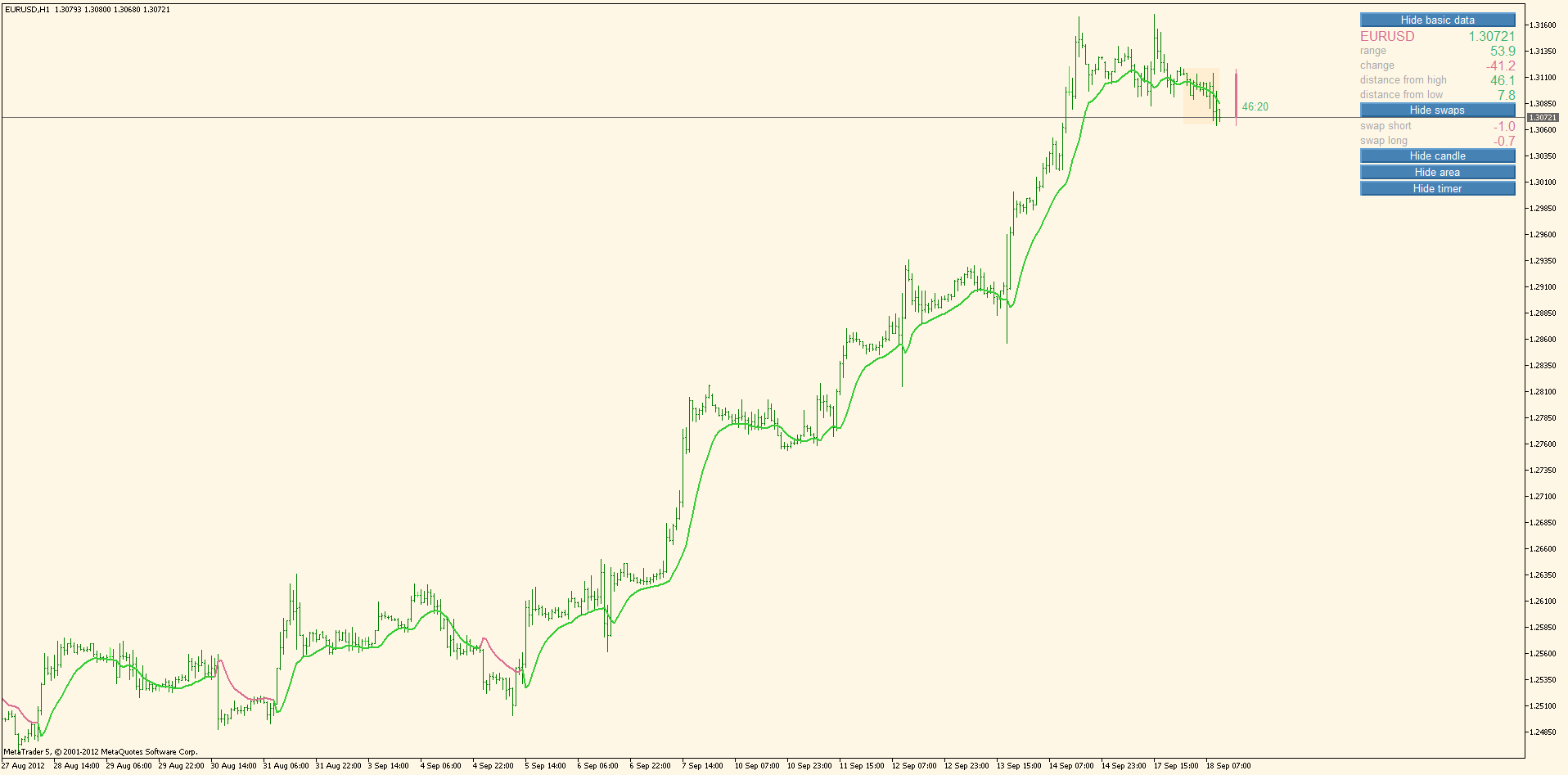Files:139679

High low activator bars ...

High low activator (the one from the previous post) bars version for metatrader 5

Files:139679

Directional RSI 2

Indicator from this post : https://www.mql5.com/en/forum/181297/page20 with some extras. Added filtering and parabolic SAR for as an estimate of trend change (instead of using levels for that purpose)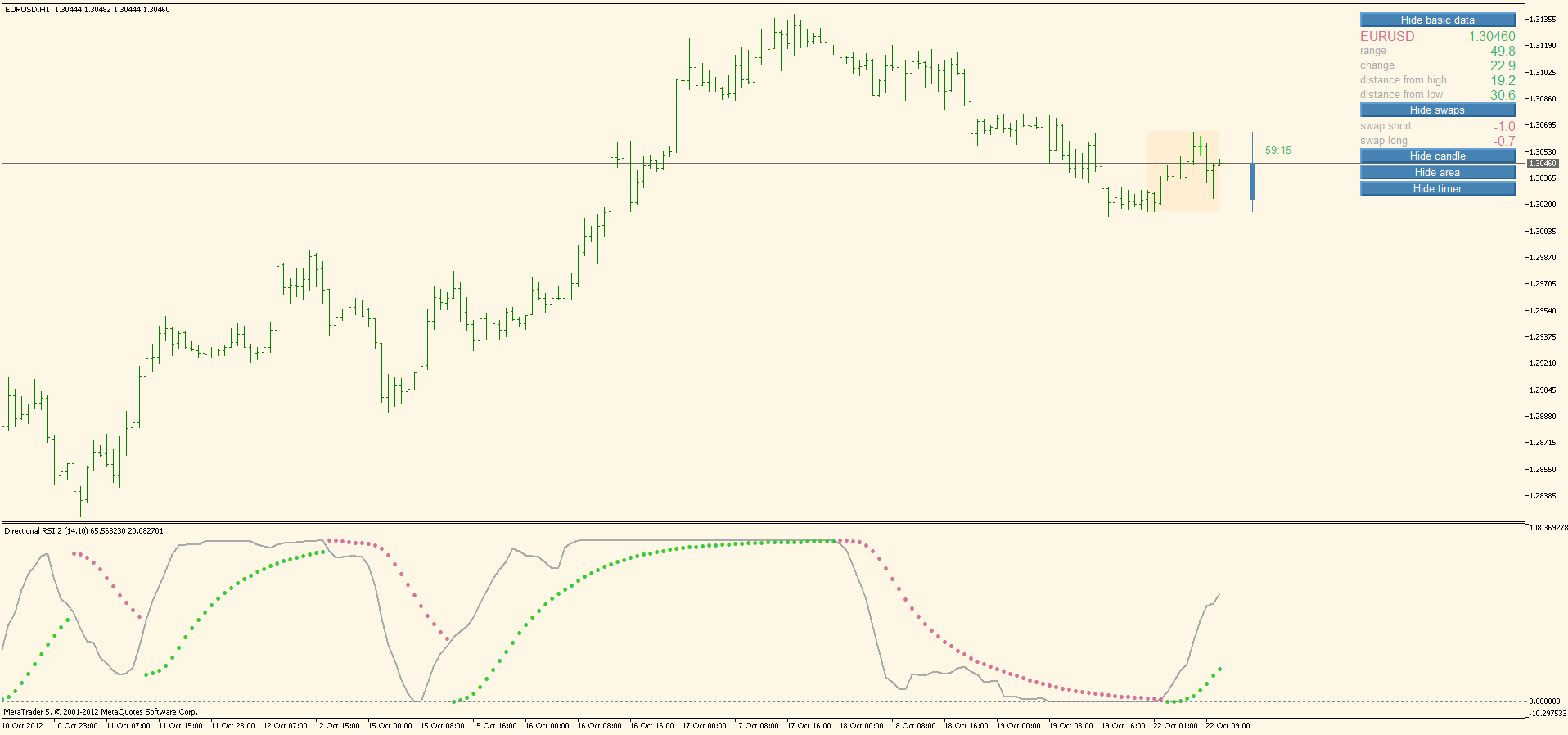Files:
drsi_2.mq5 8 kb
5_drsi_2.png 78 kb139679

Multi pass averages

Multi pass averages metatrader 5 version (it is similar to multi weighted MA but this one allows the additional 16 types of averages instead of using just LWMA). As usual, this metatrader 5 version is made so that it can be applied to other indicators too (it can accept other indicator data in the price field). Maximal depth is up to 25. An example of repeatedly applied multi pass average to each other (previous indicator data)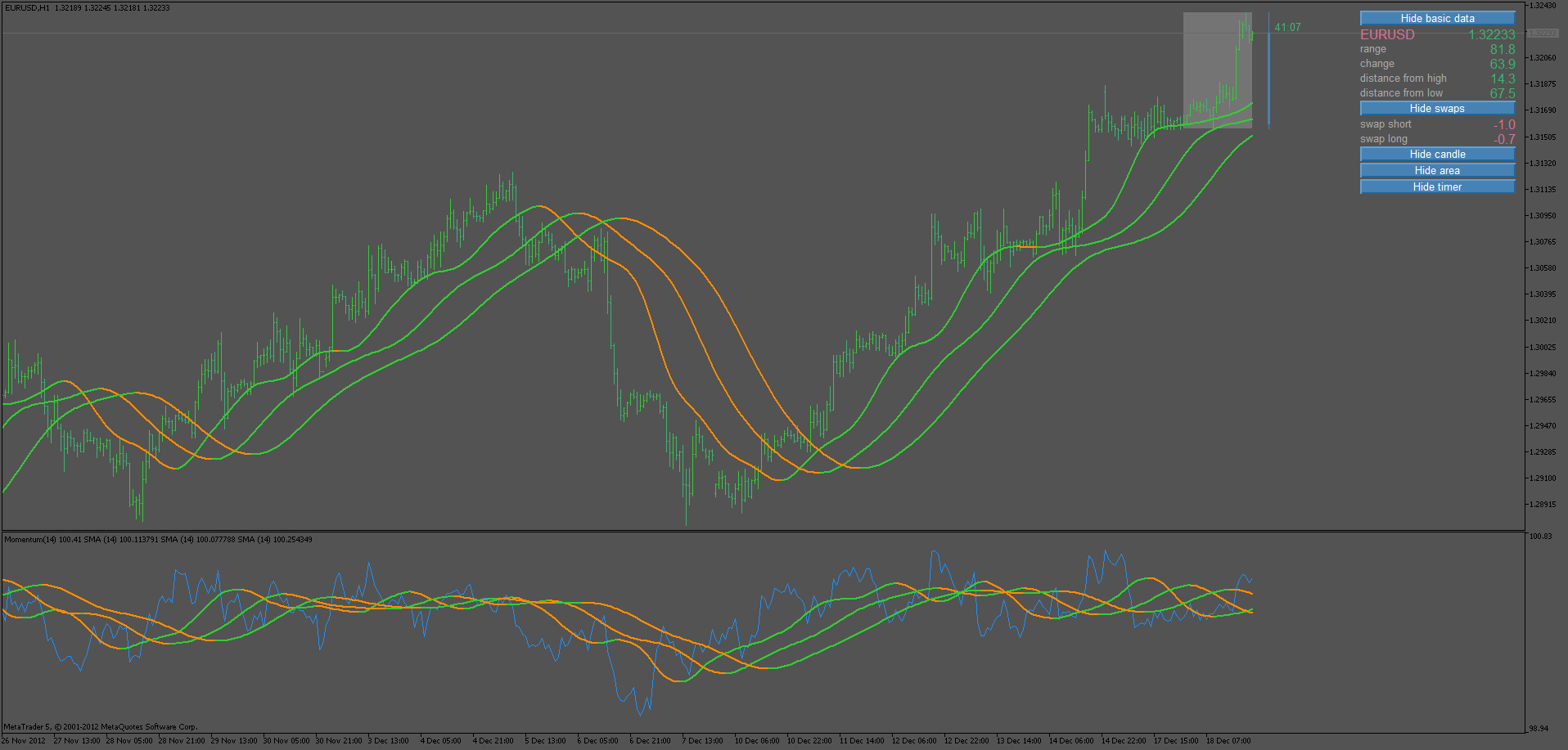Files:139679

DSS of averages ...

DSS of averages indicator with our usual choice of 18 types of averages also made show gradient colors instead of just 2 colors. The averages that can be used are our usual following 18 types:

0 = SMA

1 = EMA

2 = Double smoothed EMA

3 = Double EMA (DEMA)

4 = Triple EMA (TEMA)

5 = Smoothed MA

6 = Linear weighted MA

7 = Parabolic weighted MA

8 = Alexander MA

9 = Volume weighted MA

10 = Hull MA

11 = Triangular MA

12 = Sine weighted MA

14 = IE/2

15 = NonLag MA

16 = Zero lag EMA

Files:139679

Absolute strength of averages

Absolute strength of averages. It is upgraded to use the possible 18 types of averages in calculation. Signal lines are left in it since I think that it is very useful to see bulls and bears trends change as well (and signal lines do exactly that (an early warning of bulls and bears trend changes) Overall trend is still the cross of the main bulls and bears trend. Averages used in it are the usual ones :

0 = SMA

1 = EMA

2 = Double smoothed EMA

3 = Double EMA (DEMA)

4 = Triple EMA (TEMA)

5 = Smoothed MA

6 = Linear weighted MA

7 = Parabolic weighted MA

8 = Alexander MA

9 = Volume weighted MA

10 = Hull MA

11 = Triangular MA

12 = Sine weighted MA

14 = IE/2

15 = NonLag MA

16 = Zero lag EMA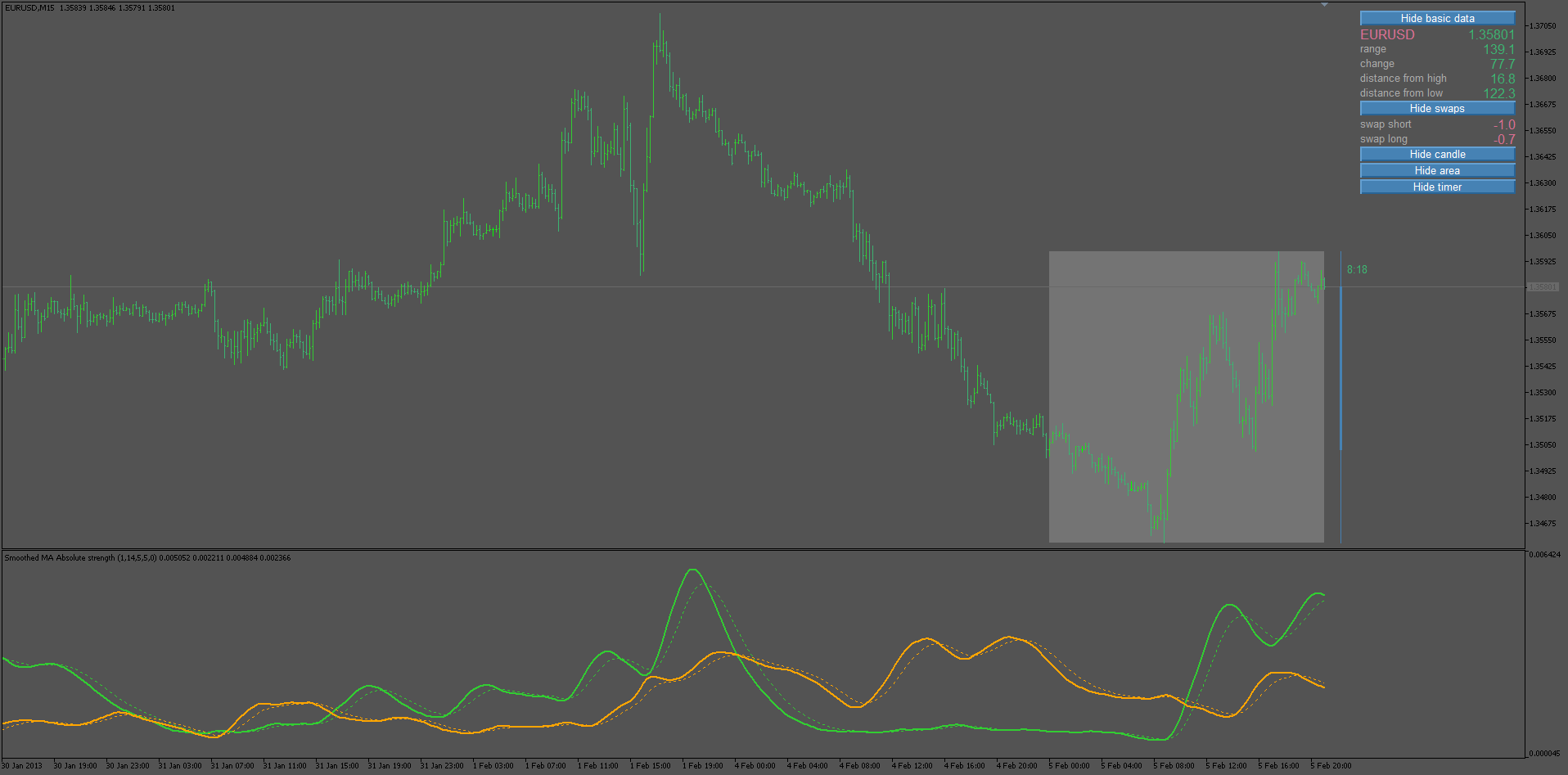Files:247

Absolute strength of averages. It is upgraded to use the possible 18 types of averages in calculation. Signal lines are left in it since I think that it is very useful to see bulls and bears trends change as well (and signal lines do exactly that (an early warning of bulls and bears trend changes) Overall trend is still the cross of the main bulls and bears trend. Averages used in it are the usual ones :
0 = SMA

1 = EMA

2 = Double smoothed EMA

3 = Double EMA (DEMA)

4 = Triple EMA (TEMA)

5 = Smoothed MA

6 = Linear weighted MA

7 = Parabolic weighted MA

8 = Alexander MA

9 = Volume weighted MA

10 = Hull MA

11 = Triangular MA

12 = Sine weighted MA

13 = Linear regression

14 = IE/2

15 = NonLag MA

16 = Zero lag EMAjust wondering if this great indi is available in MT4 version? I haven't been able to locate it.

thanks very much for all your help.

David417